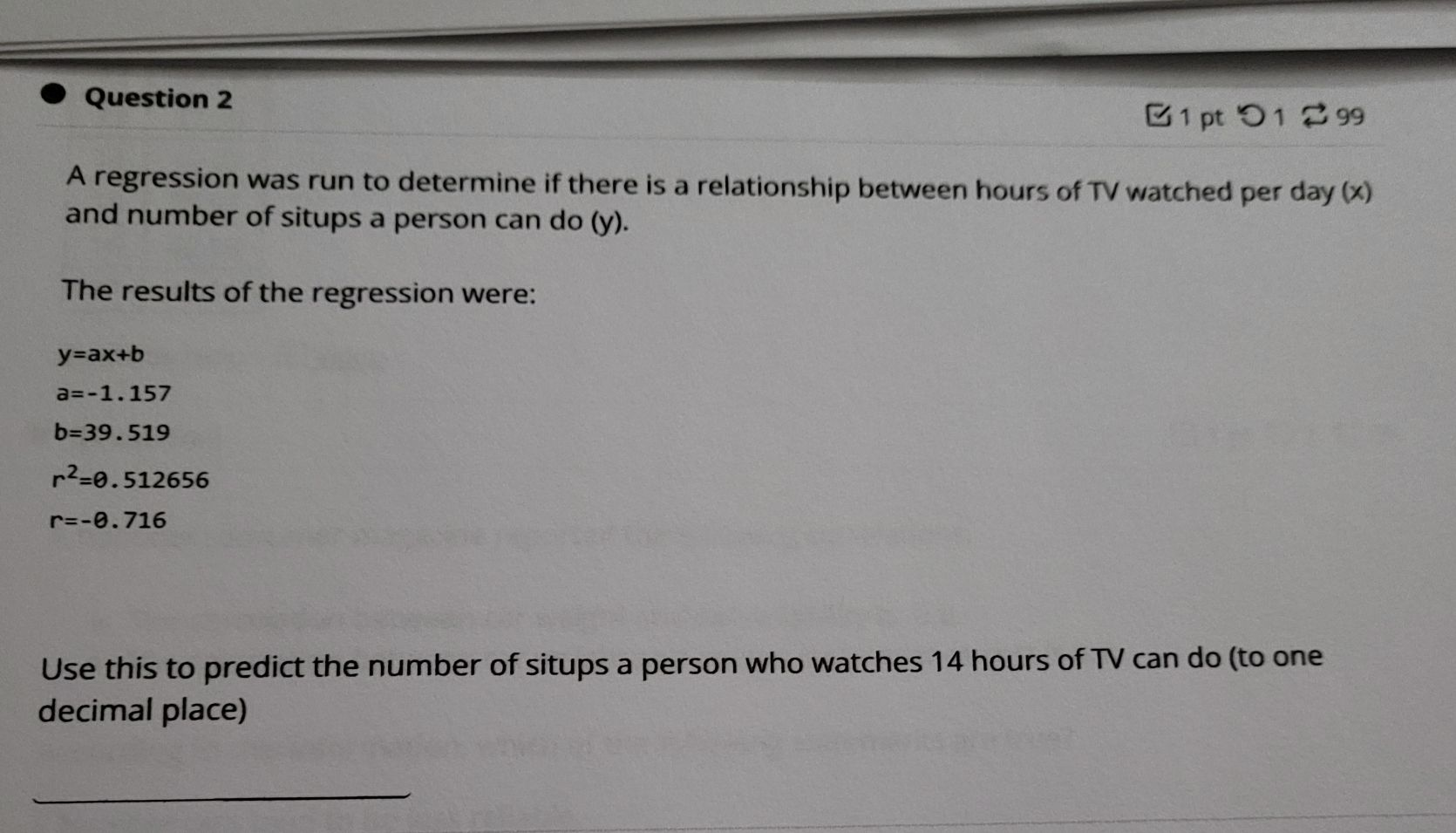### Still have math questions?

Algebra
QuestionQuestion $$2$$ A regression was run to determine if there is a relationship between hours of TV watched per day $$( x )$$ and number of situps a person can do $$( y )$$ . The results of the regression were:

$$y = a x + b$$

$$a = - 1.157$$

$$b = 39.519$$

$$r ^ { 2 } = 0.512656$$

$$r = - 0.716$$